# Bihar Board Class 10 Science Solutions Chapter 12 Electricity

BSEB Bihar Board Class 10 Science Solutions Chapter 12 Electricity Textbook Questions and Answers.

## Bihar Board Class 10 Science Solutions Chapter 12 Electricity

### Bihar Board Class 10 Science Chapter 12 Electricity InText Questions and Answers

In-text Questions (Page 200)

Question 1.
What does an electric circuit mean?
A continuous conducting path consisting of wires and other resistances (like electric bulb, etc.) and a switch, between the two terminals of a cell or battery, along which an electric current flows, is called a circuit.

Free Median Calculator – find the Median of a data set step-by-step.

Question 2.
Define the unit of current.
The SI unit of electric current is ampere (which is denoted by the letter A). When 1 coulomb of charge flows through any cross-section of a conductor in 1 second, the current flowing through it is said to be 1 ampere.

Question 3.
Calculate the number of electrons constituting one coulomb of charge.
The charge on an electron, e = 1.6 × 10-19 c. No. of electrons constituting 1 coulomb is given by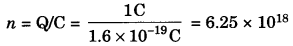In-text Questions (Page 202)

Question 1.
Name a device that helps to maintain a potential difference across a conductor.
An electrical cell (or a battery).

Question 2.
What is mean by saying that the potential difference between two points is 1 V?
The potential difference between two points is said to be 1 volt (1 V) if 1 joule of work is done in moving 1 coulomb of electric charge from one point to the other.

Question 3.
How much energy is given to each coulomb of charge passing through a 6 V battery?
W = QV = 1C × 6V = 6J

In-text Questions (Page 209)

Question 1.
On what factors does the resistance of a conductor depend?
The electrical resistance of a conductor (or a wire) depends on the following factors:

• length of the conductor,
• area of cross-section of the conductor (or thickness of the conductor),
• nature of the material of the conductor, and
• temperature of the conductor.

Question 2.
Will current flow more easily through a thick wire or a thin wire of the same material, when connected to the same source? Why?
The current will flow more easily through a thick wire than through a thin wire of the same material when connected to the same source (like a battery). This is due to the fact that the resistance of a wire is inversely proportional to the square of its diameter. A thick wire has greater diameter and hence lesser resistance making the current to flow through it more easily. On the other hand, a thin wire has smaller diameter and hence greater resistance to the flow of current through it.

Question 3.
Let the resistance of an electrical component remain constant while the potential difference across the two ends of the component decreases to half of its former value. What change will occur in the current through it?
The current through the component will also decrease to half of its former value.

Question 4.
Why are coils of electric toasters and electric irons made of an alloy rather than a pure metal?
The coils (or heating elements) of toasters and electric irons are made of an alloy rather than a pure metal because:

• the resistivity of an alloy is much higher than that of a pure metal, and
• an alloy does not undergo oxidation (or burn) easily even at high temperature, when it is red hot.

Question 5.
Use the data in Table on page 207 of this book to answer the following:
(a) Which among iron and mercury is a better conductor?
(b) Which material is the best conductor?
(a) The electrical resistivity of iron is 10.0 × 10-8 Ω m whereas that of mercury is 94.0 × 10-8 Ω m. Since the resistivity of iron is less than that of mercury, therefore, iron is a better conductor than mercury.
(b) Silver metal has the lowest electrical resistivity (1.60 × 10-8 Ω m), therefore, silver metal is the best conductor of electricity.

In-text Questions (Page 213)

Question 1.
Draw a schematic diagram of a circuit consisting of a battery of three cells of 2 V each, a 5 Ω resistor, an 8 Ω resistor, and a 12 Ω, resistor, and a plug key, all connected in series.
A schematic diagram of a circuit consisting of a battery of three 2V cells, a 5Ω resistor, an 8Ω resistor and a 12Ω resistor and a plug key, K; all connected in series, is shown in figure given below.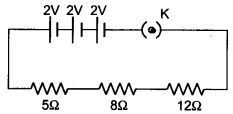Question 2.
Redraw the circuit of Question 1 putting an ammeter to measure the current through the resistors and a voltmeter to measure the potential difference across the 12 Ω resistor. What would be the reading in the ammeter and the voltmeter?
The circuit diagram of question 1 with an ammeter and voltmeter V across the 12 Ω resistor is shown in figure given below.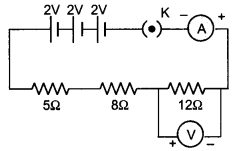Since three resistance are in series.
Total resistance of circuit, R = 5Ω + 8Ω + 12Ω = 25Ω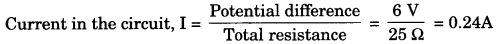Potential difference across 12 Ω resistor i.e. V = current × resistance = 0.24 × 12 = 2.88V
Reading of ammeter is 0.24 A and reading of voltmeter is 2.88 V.

In-text Questions (Page 216)

Question 1.
Judge the equivalent resistance when the following are connected in parallel:
(a) 1 Ω and 106 Ω
(b) 1 Ω, 103 Ω and 106 Ω
(a) The equivalent resistance of two resistances 1 Ω and 106 Ω connected in parallel will be less than 1 Ω. This is because when a number of resistances are connected in parallel, then their equivalent resistance is less than the smallest individual resistance (which is 1 Ω in this case).
(b) The equivalent resistance of three resistances 1 Ω,103 Ω and 106 Ω connected in parallel will be less than 1 Ω.

Question 2.
An electric lamp of 100 Ω, a toaster of resistance 50 Ω, and a water filter of resistance 500 Ω are connected in parallel to a 220 V source. What is the resistance of an electric iron connected to the same source that takes as much current as all three appliances, and what is the current through it?
The combined resistance R of three resistors (or electrical devices) R1, R2 and R3, connected in parallel is given by the formula:
$$\frac{1}{R}=\frac{1}{R_{1}}+\frac{1}{R_{2}}+\frac{1}{R_{3}}$$
Here, Resistance of electric lamp, R1 = 100 Ω
Resistance of toaster, R2 = 50 Ω
and, Resistance of water filter, R3 = 500 Ω
Putting these values of R1, R2 and R3 in the above formula, we get: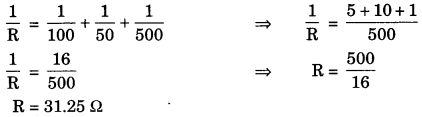Thus, the resistance of electric iron which takes the same current as drawn by all the three appliances will be 31.25 Ω.
Now, Potential difference, V = 220 V
Current, I = ? (To be calculated)
and, Resistance, R = 31.25 Ω (Calculated above)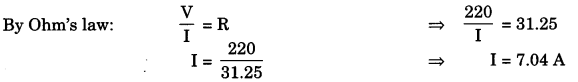Thus, the current passing through the electric iron is 7.04 amperes.

Question 3.
What are the advantages of connecting electrical devices in parallel with the battery instead of connecting them in series?
The advantages of connecting electrical devices in parallel are the following:
(i) In parallel circuits, if one electrical appliance stops working due to some defect, even then all other appliances keep working normally. On the other hand, in a series circuit, if one electrical appliance stops working due to some defect, then all the other appliances also stop working (because the whole circuit is broken).

(ii) In parallel circuits, each electrical appliance has its own switch due to which it can be turned on or off independently, without affecting the other appliances. In a series circuit, all the electrical appliances have only one switch due to which they cannot be turned on or off independently.

(iii) In parallel circuits, each electrical appliance gets the same voltage as that of the battery due to which all the appliances work properly. In a series circuit, the appliances do not get the same voltage as that of the battery because the voltage is shared by all the appliances.

(iv) In the parallel connection of electrical appliances, the overall resistance of the circuit is reduced due to which the current from the battery is high and hence each electrical appliance can draw the required amount of current. In the series connection of electrical appliances, the overall resistance of the circuit cannot draw sufficient current for their proper working.

Question 4.
How can three resistors of resistances 2 Ω, 3 Ω, and 6 Ω be connected to give a total resistance of (a) 4 Ω, and (b) 1 Ω ?
(a) In order to obtain a total resistance 4 Ω from three resistors of 2 Ω, 3 Ω and 6 Ω:
(i) First connect the two resistors of 3 Ω and 6 Ω in parallel to get a total resistance of 2 Ω. This is because in parallel combination: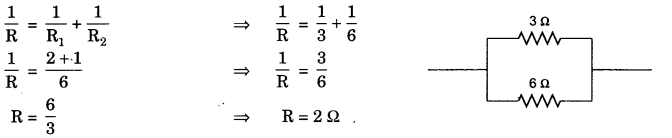(ii) Then the parallel combination of 3 Ω and 6 Ω resistors (which is equivalent to 2 Ω resistance) is connected in series with the remaining 2 Ω resistor to get a total resistance of 4 Ω. This is because in series combination:
R = R1 + R2
R = 2 + 2
R = 4Ω
The arrangement of three resistors of 2 Ω, 3 Ω and 6 Ω which gives a total resistance of 4 Ω can now be represented as follows: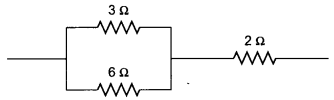Thus, we can obtain a total resistance of 4 Ω by connecting a parallel combination of 3 Ω and 6 Ω resistors in series with 2 Ω resistor.

(b) In order to obtain a total resistance of 1 Ω from the three resistors of 2 Ω, 3 Ω and 6 Ω, all the three resistors should be connected in parallel. This is because in parallel combination: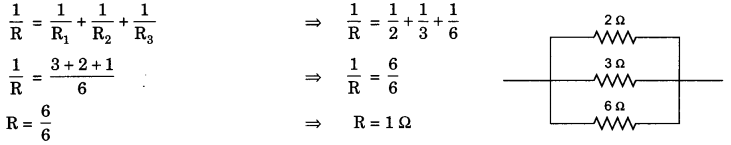Question 5.
What is (a) the highest, and (b) the lowest, total resistance that can be secured by the combination of four coils of resistance 4 Ω, 8 Ω,12 Ω and 24 Ω ?
(a) The highest resistance can be secured (or obtained) by connecting all the four coils in series. In this case:
R = R1 + R2 + R3 + R4
R = 4 + 8 + 12 + 24
R = 48 Ω
Thus, the highest resistance which can be secured is 48 ohms.

(b) The lowest resistance can be secured by connecting all the four coils in parallel. In this case: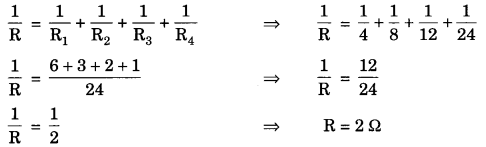Thus, the lowest resistance which can be secured is 2 ohms.

In-text Questions (Page 218)

Question 1.
Why does the cord of an electric heater not glow while the heating element does?
The heating element of an electric heater is made of an alloy (such as nichrome) which has high resistance whereas the cord is made of copper metal which has very, very low resistance. The heating element glows because it becomes red-hot due to the large amount of heat produced on passing current (because of its high resistance). On the other hand, the connecting cord of the electric heater made of copper does not glow because negligible heat is produced in it by passing the same current (due to its extremely low resistance).

Question 2.
Compute the heat generated while transferring 96000 coulomb of charge in one hour through a potential difference of 50 V.
First of all we will calculate the current (I) by using the given values of charge (Q) and time (t). We know that:
Current, I = $$\frac{\mathrm{Q}}{t}=\frac{9600 \mathrm{C}}{1 \mathrm{~h}}=\frac{96000 \mathrm{C}}{60 \times 60 \mathrm{~s}}$$
or, I = 26.67 A …………(1)
We will now calculate the resistance using Ohm’s Law
Resistance, R = $$\frac{V}{I}=\frac{50 \mathrm{~V}}{26.67 \mathrm{~A}}$$
or R = 1.87 Ω …….(2)
and, Time, t = 1h = 60 × 60s = 3600 s ……(3)
Heat generated, H = I2 × R × t = (26.67)2 × 1.87 × 3600 J = 4788397 J = 4.79 × 106 J or 4.8 × 106 J
Thus, the heat generated is 4.8 × 106 joules.

Question 3.
An electric iron of resistance 20 Ω takes a current of 5 A. Calculate the heat developed in 30 s.
Here, Current, I = 5 A
Resistance, R = 20 Ω
and, Time, t = 30 s
Now, Heat produced, H = I2 × R × t ⇒ H = (5)2 × 20 × 30
H = 25 × 20 × 30 ⇒ H = 15000 J
Thus, the heat developed is 15000 joules.

In-text Questions (Page 220)

Question 1.
What determines the rate at which energy is delivered by a current?
Electric power of the appliance.

Question 2.
An electric motor takes 5 A from a 220 V line. Determine the power of the motor and the energy consumed in 2 h.
Here, Voltage, V = 220 V and, Current, I = 5 A
Now, Power, P = V × I
So, P = 220 × 5 W
∴ P = 1100 W
Thus, the power of the motor is 1100 watts.
Now, Power, P = 1100 W (Calculated above)
Time, t = 2h = 2 × 60 × 60 s = 7200 s
So, Energy, E = P × t
E = 1100 × 7200 J
E = 7.92 × 106 J
Thus, the energy consumed is 7.92 × 106 joules.

### Bihar Board Class 10 Science Chapter 12 Electricity Textbook Questions and Answers

Question 1.
A piece of wire of resistance R is cut into five equal parts. These parts are then connected in parallel. If the equivalent resistance of this combination is R’, then the ratio R/R’ is:
(a) 1/25
(b) 1/5
(c) 5
(d) 25
After cutting the wire, resistance of each part = $$\frac{\mathrm{R}}{5}$$
Equivalent resistance when these parts connected in parallel.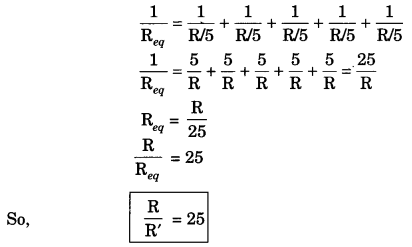Question 2.
Which of the following terms does not represent electrical power in a circuit?
(a) I2R
(b) IR2
(c) VI
(d) V2/R
Electric power
P = VI = (IR) I = I2R = $$\left(\frac{\mathrm{V}^{2}}{\mathrm{R}^{2}}\right) \mathrm{R}=\frac{\mathrm{V}^{2}}{\mathrm{R}}$$
So, IR2 does not represent electric power in circuit.

Question 3.
An electric bulb is rated 220 V and 100 W. When it is operated on 110 V, the power consumed will be
(a) 100 W
(b) 75 W
(c) 50 W
(d) 25 W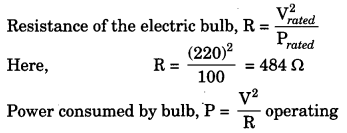Here, operating voltage = 110 V
P = $$\frac{(110)^{2}}{484}$$ = 25 W

Question 4.
Two conducting wires of the same material and of equal lengths and equal diameters are first connected in series and then parallel in a circuit across the same potential difference. The ratio of heat produced in series and parallel combinations would be
(a) 1: 2
(b) 2 : 1
(c) 1 : 4
(d) 4 : 1
Since both wires are made up of same material and all other parameters are identical,
so, resistance of both the wires is same. Let it be R. When connected in series, their equivalent resistance is given by
Req = R1 + R2 = R + R = 2R
When connected in parallel, their equivalent resistance is given by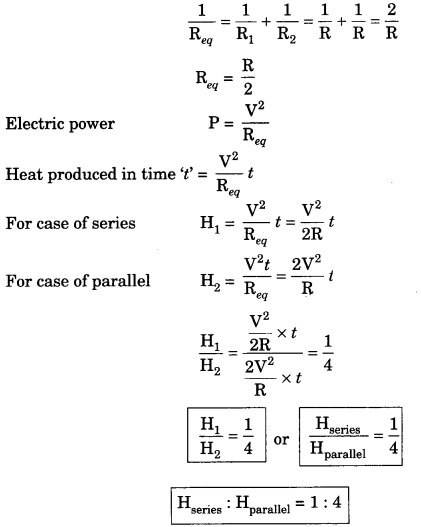Question 5.
How is a voltmeter connected in the circuit to measure the potential difference between two points?
A voltmeter is always connected in parallel in the circuit across the points between which the potential difference is to be determined.

Question 6.
A copper wire has diameter 0.5 mm and resistivity of 1.6 × 10-8 Ω m. What will be the length of this wire to make its resistance 10 Ω? How much does the resistance change if the diameter is doubled?
Given, diameter of wire, D = 0.5 mm = 0.5 × 10-3 m
Resistivity of copper, ρ = 1.6 × 10-8 Ω m
Resistance = 10 Ω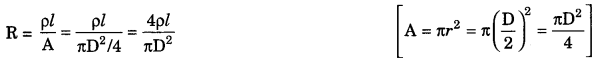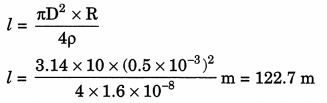As R ∝ $$\frac{1}{r^{2}} \propto \frac{1}{D^{2}}$$, when D doubled, resistance becomes $$\frac {1}{4}$$ times.

Question 7.
The values of current I flowing in a given resistor for the corresponding values of potential difference V across the resistor are given below: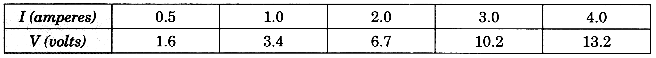Plot a graph between V and I and calculate the resistance of that resistor.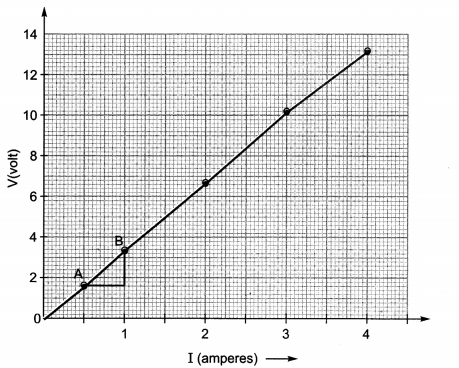Taking two points A and B
A(0.5 A, 1.6 V) and B (1.0 A, 3.4 V)
Slope = $$\frac{\Delta \mathrm{V}}{\Delta \mathrm{I}}$$
Here, ΔV = 1.8 V, ΔI = 0.5 A
R = $$\frac{\Delta \mathrm{V}}{\Delta \mathrm{I}}=\frac{1.8 \mathrm{~V}}{0.5 \mathrm{~A}}$$ = 3.6 Ω

Question 8.
When a 12 V battery is connected across an unknown resistor, there is a current of 2.5 mA in the circuit. Find the value of the resistance of the resistor.
Here V = 12 V, I = 2.5 mA = 2.5 × 10-3 A
Resistance = $$\frac{V}{I}=\frac{12 V}{2.5 \times 10^{-3} \mathrm{~A}}$$ = 4800 Ω = 4.8 kΩ

Question 9.
A battery of 9 V is connected in series with resistors of 0.2 Ω, 0.3 Ω, 0.4 Ω, 0.5 Ω and 12 Ω, respectively. How much current would flow through the 12 Ω resistor?
Equivalent resistance = 0.2 Ω + 0.3 Ω + 0.4 Ω + 0.5 Ω + 12 Ω
= 13.4 Ω
Current through circuit, I = $$\frac{\mathrm{V}}{\mathrm{R}_{e q}}=\frac{9 \mathrm{~V}}{13.4 \Omega}$$ = 0.67 A
In series, the current flowing through all resistor is same. Thus, current flowing through 12 Ω resistor = 0.67 A.

Question 10.
How many 176 Ω resistors (in parallel) are required to carry 5 A on a 220 V line?
Given, I = 5 A, V = 220 V
Resistance of the circuit, R = $$\frac{\mathrm{V}}{\mathrm{I}}=\frac{220 \mathrm{~V}}{5 \mathrm{~A}}$$ = 44 Ω
Resistance of each resistor, r = 176 Ω
In case of parallel combination of identical resistors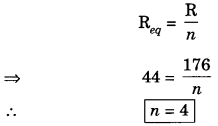Number of resistors required = 4

Question 11.
Show how you would connect three resistors, each of resistance 6 Ω, so that the combination has a resistance of (i) 9 Ω (ii) 4 Ω.
1. For getting equivalent resistance Req = 9Ω two resistors will be connected in parallel and the third resistors will be connected in series with this parallel combination.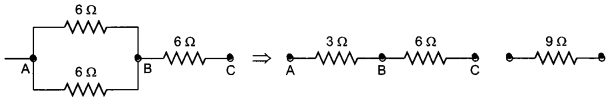2. For getting equivalent resistance Req = 4 Ω two resistors should be connected in series. The combination of which will lead to equivalent of 12 Ω afterwards connecting this 12 Ω resistor with 6 Ω resistor in parallel, the overall equivalent resistance will be 4 Ω.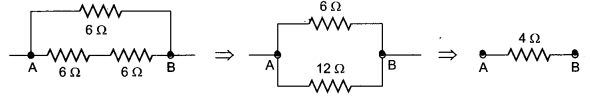Question 12.
Several electric bulbs designed to be used on a 220 V electric supply line, are rated 10 W. How many lamps can be connected in parallel with each other across the two wires of 220 V line if the maximum allowable current is 5 A?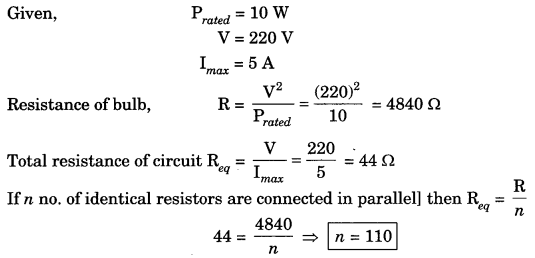Question 13.
A hot plate of an electric oven connected to a 220 V line has two resistance coils A and B, each of 24 Ω resistance, which may be used separately, in series, or in parallel. What are the currents in the three cases?
Ans. Given, potential difference = 220 V
Resistance of each coil RA = RB = 24 Ω
(i) When each coil A or B is connected separately, current through each coil i.e.,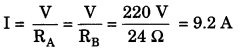(ii) When coil A and B connected in parallel equivalent resistance in the circuit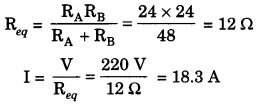(iii) When coil A and B are connected in series equivalent resistance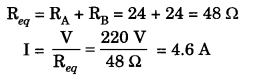Question 14.
Compare the power used in the 2 Ω resistor in each of the following circuits: (i) a 6 V battery in series with 1 Ω and 2 Ω resistors, and (ii) a 4 V battery in parallel with 12 Ω and 2 Ω resistors.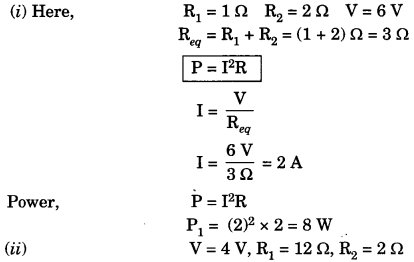In a parallel circuit, potential (V) across each resistor is the same and is equal to the potential applied across the circuit.
Power dissipation through 2 Ω resistor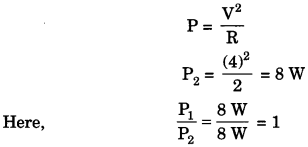Question 15.
Two lamps, one rated 100 W at 220 V, and the other 60 W at 220 V, are connected in parallel to electric mains supply. What current is drawn from the line if the supply voltage is 220 V?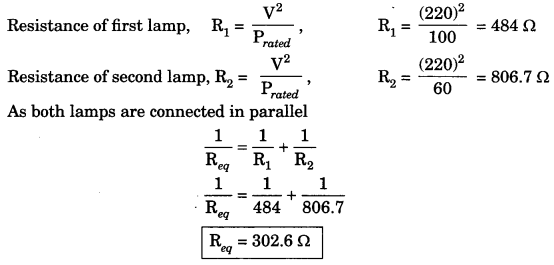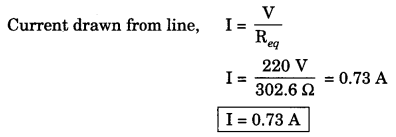Question 16.
Which uses more energy, a 250 W TV set in 1 hour, or a 1200 W toaster in 10 minutes?
Energy used by 250 W TV set in 1 hour = (250 W) (1 h) = 250 Wh
Energy consumed by 1200 W toaster in 10 min = $$\left(\frac{1}{6} h\right)$$ = 1200 W × $$\frac {1}{6}$$ h = 200 Wh
Energy consumed by 1200 W toaster in 10 minutes is less than energy consumed by 250 W TV set in 1 hour.
Hence energy consumed by the TV set is more.

Question 17.
An electric heater of resistance 8 Ω draws 15 A from the service mains for 2 hours. Calculate the rate at which heat is developed in the heater.
Given, I = 15A, R = 8Ω, t = 2h
Rate at which heat is developed i.e., electric power
P = I2R = (15)2 × 8 = 1800 W = 1800 J/S.

Question 18.
Explain the following:
(а) Why is the tungsten used almost exclusively for filament of electric lamps?
(b) Why are the conductors of electric heating devices, such as bread-toasters and electric irons, made of an alloy rather than a pure metal?
(c) Why is the series arrangement not used for domestic circuits?
(d) How does the resistance of a wire vary with its area of cross-section?
(e) Why are copper and aluminium wires usually employed for electricity transmission?
(a) Tungsten has high melting point (3380°C). It does not melt and becomes very hot emitting light.
(b) The resistivity of an alloy is generally higher than that of pure metals and alloys do not oxidise readily at high temperatures.
(c) In series arrangement, if any one of the appliances fails or is switched off, all the other appliances stop working.
(d) Resistance of a wire is inversely proportional to its area of cross-sectional area (A)
R ∝ $$\frac{1}{\mathrm{~A}}$$
(e) Copper and aluminium posses low resistivity and hence low energy losses.

### Bihar Board Class 10 Science Chapter 12 Electricity Textbook Activities

Activity 12.1 (NCERT Textbook Page 203-204)

Discussion and Conclusion:
The graph of V and I is a straight line passing through origin and slope of graph is equal to resistance

Activity 12.2 (NCERT Textbook Page 205)

Discussion and Conclusion:
The readings of ammeter are different for different components. This is due to the reason that resistances of all the components are different.

Activity 12.3 (NCERT Textbook Page 206)

Discussion and Conclusion:

• When the length of a wire is doubled, the resistance of the wire becomes twice as R ∝ l and current becomes half as I = $$\frac{\mathrm{V}}{\mathrm{R}}$$
• When the wire is replaced by a thicker nichrome wire, the resistance of wire decreases as R ∝ $$\frac{1}{\mathrm{~A}}$$ and current increases as I = $$\frac{\mathrm{V}}{\mathrm{R}}$$
• When the nichrome wire is replaced by a copper wire of same length and cross-section, current decreases as resistivity of nichrome is greater as R ∝ p.
• Resistance of a wire depends on the resistivity of the material, length of wire and area of cross-section. R = ρ$$\frac{l}{\mathrm{~A}}$$

Activity 12.4 (NCERT Textbook Page 210)

Discussion and Conclusion:

• Here total resistance, R = 1Ω + 2Ω + 3Ω = 6Ω, Reading of ammeter is found to be around 6V/6Ω = 1A.
• When the position of the ammeter is changed, the reading remains the same.

Activity 12.5 (NCERT Textbook Page 211)

Discussion and Conclusion:

• Potential difference across the series combination is equal to potential difference across the two terminals of the battery.
• Relation between potential drop across battery and resistors V = V1 + V2 + V3.

Activity 12.6 (NCERT Textbook Page 213-214)

Discussion and Conclusion:

• Potential difference across each resistor is same.
• Net current is equal to the sum of current through each resistor.